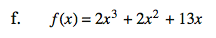Home > CCA2 > Chapter 10 > Lesson 10.2.2 > Problem10-128

10-128.
1. Without graphing, find where each of the following curves crosses the x-axis. (Find the exact points!) Homework Help ✎

1. f(x) = x2x − 12

2. f(x) = 2x2 − 3x − 9

3. f(x) = 2x2 + x – 7

4. f(x) = 3x2 – 2x + 7

5. f(x) = 3x3 + 2x2 – 8x

6. f(x) = 2x3 + 2x2 + 13x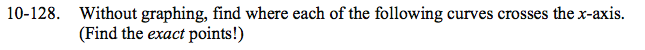For each equation, let f(x) = 0. Then solve by factoring and/or using the Quadratic Formula.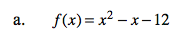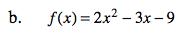x = −1.5, 3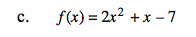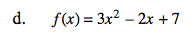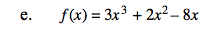Each term has an x.

$x=0,-2,\frac{4}{3}$